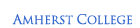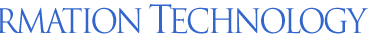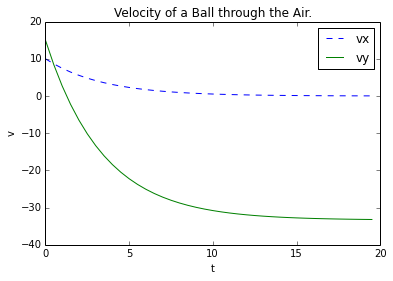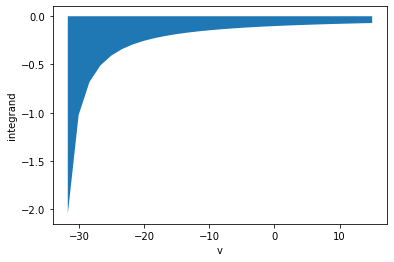ATS > Software > Scientific Programming with Python > Newton’s Laws of Motion# Scientific Programming with Python

## Part 2: Newton’s Laws of Motion

#### Next: Simulating a Magnet

The laws of motion are often far more complicated than the simple law of gravity.

## The Force of Gravity

Constant gravitational acceleration was established by Galileo, but Newton provided the more general form.

### Acceleration and Force

Recall the law of gravity near the Earth’s surface, describing the position of an object travelling vertically:

y = y0 + vy0t − ½ gt2

If we take the derivative of this expression we get one for the velocity:

v = dy/dt = vy0gt

which shows how it becomes more and more negative with time in response to the downward acceleration of gravity.

If we take another derivative we get an expression for the acceleration:

a = dv/dt = −g

We can write this in vector notation using bold face to represent vector quantities, for example g = (0, −g) since it’s a constant value pointing downward:

a = dv/dt = g

Experimental examination of gravity and other forces in nature such as friction tell us that the important kinematic quantity here is the vector acceleration, which is zero except in the presence of such forces:

m a = Σi Fi

where m is the mass of the moving object. This is Newton’s second law of motion.

The force of gravity is therefore

FG = m g

## The Force of Air Resistance

Air resistance is a common force that is non-constant, and works against gravity to slow you down.

### Air Resistance, Analytically

Another force experienced by an object moving through the air is air resistance, which can be approximated by

FD = −½ ρACD|v|v

The minus sign indicates that the force is opposite to the direction of the velocity; ρ is the density of air, 1.225 kg/m3 at sea level, A is the projected area of the object perpendicular to the velocity (i.e. πr2 for a sphere), and CD is a dimensionless quantity known as the the drag coefficient.

An object traveling through the air therefore has an acceleration given by

m a = m g − ½ ρACD|v|v

From this expression we can see that a larger cross-sectional area increases the force of air resistance, so that a feather will drop more slowly than a hammer — but not on the Moon, where there is no air!

At low speeds CD ~ b/|v|, so the magnitude of the force varies directly with speed, while at high speeds CD is approximately constant (in the range of 0.1 – 1) and the magnitude of the force varies as the square of the speed. The former is the result of the displacement of air mass by the object with laminar (smooth) flow of air around it; the latter reflects turbulent flow produced by friction around the ball.

A ball thrown through the air, which has relatively low speed, therefore has an acceleration given by

dv/dt = g − ½ (ρAb/m)v

The factor −½ (ρAb/m) has units of inverse time, so we can define a characteristic time:

τ = 2m/(ρAb)

and

dv/dt = gv/τThis differential equation can be integrated relatively easily:

v0v dv/(gv/τ) = ∫0t dt

−τ ln(vgτ)|v0v = t

v = gτ + (v0gτ)e-t/τ

The transient exponential term will go away in several characteristic times, and the ball will eventually reach a terminal velocity vf = gτ. The greater the density of air and/or the projected area of the ball, the smaller the terminal velocity. Conversely, the greater the ball’s mass the greater its terminal velocity.

A typical value of b = 17 m/s, and for a baseball, A = π (3.7 cm)2 = 0.0043 m2, m = 0.15 kg, then τ = 3.4 s, so the terminal velocity is –33 m/s.

For a human in free fall, the terminal speed will be 50 m/s to 90 m/s, depending on how they hold themselves (flat or bullet-like).

We can now define a drag velocity function for any given time and initial velocity:

import numpy as np

g = 9.81
tau = 3.4
vf = g * tau

def vd(t, v0):
ext = np.exp(-t/tau)
(vx0, vy0) = v0
return np.array( (t, vx0 * ext, -vf + (vy0 + vf)*ext) )

As usual, there’re a few things going on in this function:

• The argument `t` can be an array of values, which when divided by `tau` will result in an array of each element divided by `tau`:
• np.array( (0, 1, 2) )/tau

⇒ array([ 0. , 0.29411765, 0.58823529])

Such element-by-element operations on an array are known as broadcasting.

• Similarly, we’re using the exponential function `exp()` from `numpy`, which operates element-by-element on arrays:
• np.exp( -np.array( (0, 1, 2) )/tau )

⇒ array([ -1. , -1.34194177, -1.80080771])

Note: there’s also an `exp()` function in the `math` module, but it will only accept scalar (non-sequence) values.

• We are using the `np.array()` function to return an array of arrays, as the three calculations in the return statement are each arrays of length n, producing a 3 x n array: `[ t, vx, vy ]`.

An array of time values can be generated with

t = np.arange(0,20,0.5)

This function is like the built-in `range()` but it will allow non-integer steps! More importantly, it returns an array of values, rather than a range, so we can hand it to `vdt()` to process :

v0 = (10, 15)
vdt = vd(t,v0)

The result is a triplet of arrays `[ t, vx, vy ]`:

print(vdt)

[[ 0.00000000e+00 5.00000000e-01 ...  1.85000000e+01  1.90000000e+01  1.95000000e+01]
[ 1.00000000e+01 8.63243197e+00 ...  4.33438100e-02  3.74162491e-02  3.22993225e-02]
[ 1.50000000e+01 8.38726154e+00 ... -3.31444153e+01 -3.31730775e+01 -3.31978199e+01]]And we’ll plot this to see what it looks like:

def vdplot(vdt):
from matplotlib.pyplot import plot, \
title, xlabel, ylabel, legend, \
show, close
(t, vdx, vdy) = vdt
plot(t,vdx, label='vx', linestyle='dashed')
plot(t,vdy, label='vy')
title('Velocity of a Ball through the Air.')
xlabel('t')
ylabel('v')
legend()
show()
close()

vdplot(vdt)

There are new python and `matplotlib` features here:

• The `plot()` functions here uses a combination of positional and keyword arguments like `label=` and `linestyle=`.

Keyword arguments don’t have to be in a particular position, unlike regular arguments like the x and y arrays, which must be first and second, respectively.
• The `label=` options for `plot()` are used by the `legend()` function to produce a box with the data names and styles.
• The `linestyle=` option lets you choose a variety of common ways to distinguish lines, such as 'solid' (the default), 'dotted', etc.
• In addition to the dashed line, `matplotlib` automatically assigns different colors to the different curves, but you can set your own with an option like `color='red'`.
• If you want to plot just the data points, use the function `scatter()`.

### Air Resistance, Numerically

Numerical integration of a differential equation is also possible with the SciPy package:

Here we’re importing the function `quad(integrand, initial, final)` which performs integration by quadrature, approximating the area under a curve by rectangles or trapezoids, as you probably learned about in calculus:

In this case we are using the integrand in the integral equation above to calculate the time t corresponding to each speed v in the y direction (Remember that in one dimension v becomes v, a signed value that is negative when an object is falling):

v0v dv/(-gv/τ) = ∫0t dt = t

Coded in a function:

g = 9.81
tau = 3.4
vf = g * tau
integrand = lambda v: -1/(g*(1 + v/vf))

The keyword `lambda` is an alternative way to define one-line functions. It’s known as an anonymous function because no name is defined for it, though it can be assigned to a name as above.

We want to integrate this function between `v0` and various values of `v` before it reaches the terminal velocity ` -vf = -g * tau`, the result being times corresponding to those velocities:

vs = ﻿vf * np.arange(v0/vf, -1, -0.05)
# starts at v = v0 and excludes -vf (why?)It’s helpful to plot this function to get an idea of its behavior:

import matplotlib.pyplot as plt
plt.plot(vs, integrand(vs))
plt.xlabel('v')
plt.ylabel('integrand')
plt.show()
plt.close()

Although the integrand is negative, integrating from a positive `v0` to a negative `v = -vf` reverses the sign to produce a positive time.

The function `quad()` can’t handle numpy arrays as input, so we’ll use a list comprehension:

events = [ quad(integrand, v0, v) + (v,) for v in vs ]

The returned values have the form `(t, error, v)` (the last one appended as a tuple). To print them more nicely, we can use a formatting string:

for event in events: print('%5.2f %5.2e %6.2f' % tuple(event))

-0.00 0.00e+00  15.00
0.12 1.32e-15  13.33
0.24 2.70e-15  11.66
0.37 4.12e-15  10.00
0.50 5.60e-15   8.33
0.64 7.14e-15   6.66
0.79 8.75e-15   4.99
0.94 1.04e-14   3.33
1.10 1.22e-14   1.66
1.26 1.40e-14  -0.00
1.44 1.60e-14  -1.67
1.62 1.80e-14  -3.34
1.82 2.02e-14  -5.00
2.02 2.24e-14  -6.67
2.24 2.49e-14  -8.34
2.48 2.75e-14 -10.01
2.73 3.03e-14 -11.67
3.00 3.33e-14 -13.34
3.30 3.66e-14 -15.01
3.62 1.03e-13 -16.68
3.98 1.58e-12 -18.34
4.38 2.45e-11 -20.01
4.83 3.97e-10 -21.68
5.36 6.92e-09 -23.35
5.98 5.74e-12 -25.02
6.73 3.65e-10 -26.68
7.71 3.62e-08 -28.35
9.09 1.14e-09 -30.02
11.45 1.92e-09 -31.69

We can see the error is very small so we can be confident in the result. Comparing to the result from the exact solution which is calculated for specific times:

# convert previous result to array and grab first column of times
ts = np.array(events)[:,0]
vdts = vd(ts,v0)
# vdts is a 3 x n array; we need n x 3 for the next step,
#
so use the array method transpose():
for vdt in vdts.transpose(): print('%5.2f %5.2f %6.2f' % tuple(vdt))

1.26 6.90   0.00
1.44 6.55  -1.67
1.62 6.21  -3.34
1.82 5.86  -5.00
2.02 5.52  -6.67
2.24 5.17  -8.34
2.48 4.83 -10.01
2.73 4.48 -11.67
3.00 4.14 -13.34
3.30 3.79 -15.01
3.62 3.45 -16.68
3.98 3.10 -18.34
4.38 2.76 -20.01
4.83 2.41 -21.68
5.36 2.07 -23.35
5.98 1.72 -25.02
6.73 1.38 -26.68
7.71 1.03 -28.35
9.09 0.69 -30.02
11.45 0.34 -31.69

The velocities in the third columns match! Remember, in the first case they were input to a calculation, in the second they were output from a calculation.

What’s the point other than confirming that integration by quadrature works? Well, now we can use the more general formula for drag which is important for large velocities:

m a = m g − ½ ρACDvv

dv/dt = g − (1 + |v|/c)v/τ

v0v dv/(g − (1 + |v|/c)v/τ) = ∫0t dt = t

The constant c = b / CD is very large; for b = 17 m/s and CD = 0.5, c = 34 m/s. This is comparable to the terminal velocity, ~gτ ~ 33 m/s, so the extra term becomes significant on that order of speed.

### Problem 4: A Situation That Requires Numerical Integration!

Calculate the more general solution for `vy` to see how different it is, using `vy0 = 15` m/s and assuming `vx0 = 0` . Note that `numpy.absolute()` exists!

m a = m g − ½ ρACD|v|v

Hint: Think about the effect of |v|/c both as the ball goes up (`v > 0`) and as it comes down (`v < 0`). What is the terminal velocity here? Note that `scipy.optimize.root()`exists and might be useful here!

### Next:Simulating a Magnet

Top of Page  | Using IT DocumentationIT Home  |  IT Site Map  |  Search IT